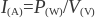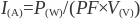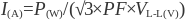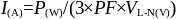# Amperage calculator Back to home

Use this calculator to determine electric current in amps (A).

## How to use an amperage calculator

1. Select current type (Direct Current, AC - Single Phase, or AC - Three Phase)
2. Enter power in watts
3. Enter voltage in volts
4. (For AC systems) Enter power factor
5. (For AC - Three Phase only) Enter voltage type: Line to Line, or Line to Neutral
6. Click on CALCULATE

### Converting Watts to Amps (Direct Current system)

Calculating current I in amps (A) can be done by dividing the power P in watts (W) by voltage V in volts (V):### Converting Watts to Amps (AC single phase system)

Determine the phase current I in amps (A) by dividing power P in watts (W) by the power factor PF multiplied by the RMS voltage V in volts (V):(The power factor is the ratio of the real power flowing to the load to the apparent power in the circuit. Power factor values can range from 0 to 1.

The RMS voltage is the square root of the mean over one cycle of the square of the instantaneous voltage.)

### Converting Watts to Amps (AC three phase system)

Line to Line Voltage:

Calculate the phase current I in amps (A) by dividing the power P in watts (W) by the square root of 3 times the power factor PF times the line to line RMS voltage VL-L in volts (V):(The power factor is the ratio of the real power flowing to the load to the apparent power in the circuit. Power factor values can range from 0 to 1.

Line to line voltage is the voltage measured between any two lines in a three phase circuit.)

Line to Neutral Voltage:

Determine the phase current I in amps (A) by dividing the power P in watts (W) by 3 times the power factor PF times the line to neutral RMS voltage VL-N in volts (V):(The power factor is the ratio of the real power flowing to the load to the apparent power in the circuit. Power factor values can range from 0 to 1.

Line to neutral voltage is the voltage measured between any line and the neutral point.)